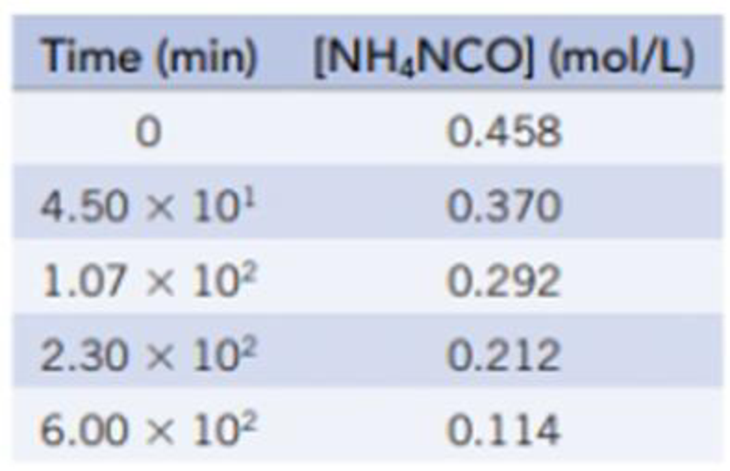Chapter 14, Problem 61GQ

Chapter
Section
Textbook Problem

Ammonium cyanate, NH4NCO, rearranges in water to give urea, (NH2)2CO.NH4NCO(aq) → (NH2)2CO(aq)Using the data in the table: (a) Decide whether the reaction is first-order or second-order. (b) Calculate k for this reaction. (c) Calculate the half-life of ammonium cyanate under these conditions. (d) Calculate the concentration of NH4NCO after 12.0 hours.

(a)

Interpretation Introduction

Interpretation: The reaction is whether first-order and second-order should be determined.

Concept introduction:

Order of a reaction:  Order of a reaction is represented by the exponential term of the respective reactant present in the rate law and the overall order of the reaction is the sum of all the exponents of all reactants present in the chemical reaction. The order of the reaction is directly proportional to the concentration of the reactants.

For a reaction,

• If the plot drawn between concentration of reactant and time is linear, then it is a zeroth order reaction
• If the plot drawn between ln[reactant] and time is linear, then it is a first order reaction
• If the plot drawn between 1/[reactant] and time is linear, then it is a second order reaction
• For the zeroth and first order reaction, the graph has a negative slope whereas the graph has a positive slope for a second order reaction.
Explanation
• Order of the reaction:

The reaction follows second order, since the graph plotted for inverse of reactant concentration with time gives a straight line whereas the graph plotted for ln of concentration values versus times does not result to a straight line graph.

Reactant concentrationTime1/(Reactant concentration)ln(Reactant concentration)0.45802.180.780.374.50×1012.70.990.2921

(b)

Interpretation Introduction

Interpretation: For the given reaction the rate constant value (k) should be determined.

Concept introduction:

Rate constant, k: The rate constant for a chemical reaction is the proportionality term in the chemical reaction rate law which gives the relationship between the rate and the concentration of the reactant present in the chemical reaction.

(c)

Interpretation Introduction

Interpretation: The half-life of ammonium cyanate should be determined.

Concept introduction:

Halflife period: It is the time required for the reactant (substrate) concentration to reduce to the one-half of its initial concentration.

The half–life period for second order reaction is as follows:

t1/2=1[A0]kWhere,k = Rate constant[A0]= initial concentrationt1/2= half-life

(d)

Interpretation Introduction

Interpretation: For the given reaction, the concentration of the reactant after 12 hours should be determined.

Concept introduction:

Rate law or rate equation: Rate law:

It is generally the rate equation that consists of the reaction rate with the concentration or the pressures of the reactants and constant parameters.

aA + bBxXRate of reaction = k [A]m[B]n

The integrated rate law for the second order is as follows:

1[A]=1[A0]+ktWhere,[A0]= initialconcentrationk=rate constantt=time[A]=concentrationaftertimet

Still sussing out bartleby?

Check out a sample textbook solution.

See a sample solution

The Solution to Your Study Problems

Bartleby provides explanations to thousands of textbook problems written by our experts, many with advanced degrees!

Get Started

Find more solutions based on key concepts